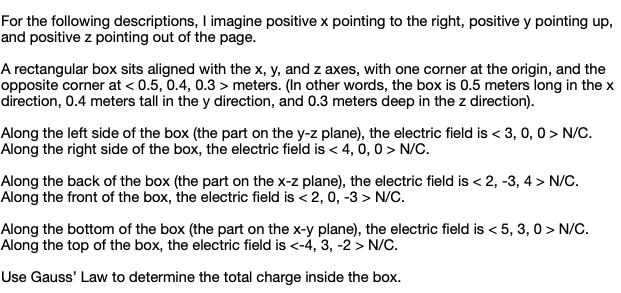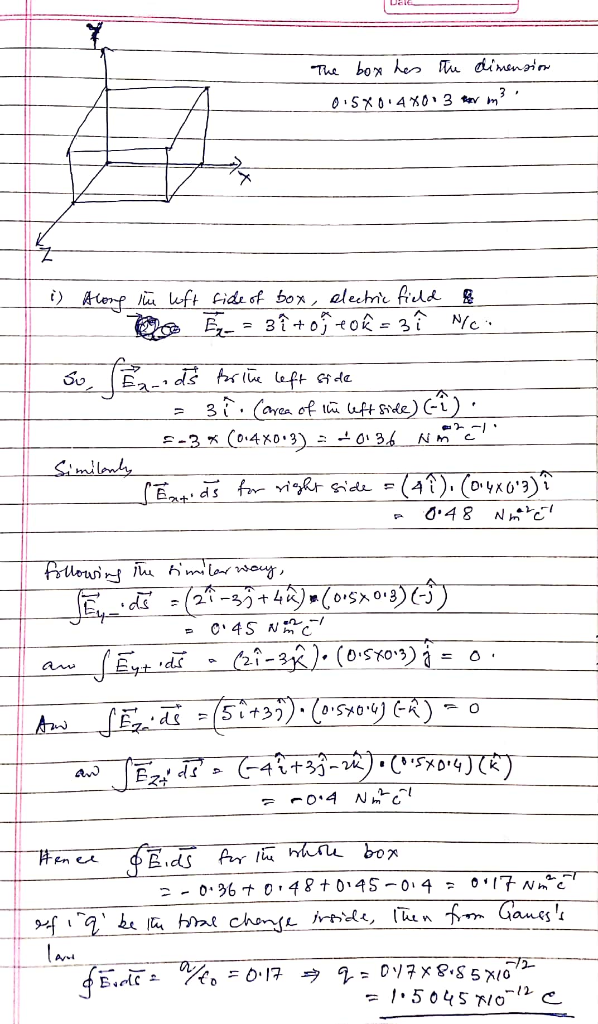Homework Help Question & Answers

And positive z pointing out of the page. A rectangular box sits aligned with the x, y, and z axes...and positive z pointing out of the page. A rectangular box sits aligned with the x, y, and z axes, with one corner at the origin, and the opposite corner at meters. (In other words, the box is 0.5 meters long in the x direction, 0.4 meters tall in the y direction, and 0.3 meters deep in the z direction). Along the left side of the box (the part on the y-z plane), the electric field is N/C. Along the right side of the box, the electric field is N/C. Along the back of the box (the part on the x-z plane), the electric field is N/C. Along the front of the box, the electric field is NC. Along the bottom of the box (the part on the x-y plane), the electric field is N/C. Along the top of the box, the electric field is N/C. Use Gauss' Law to determine the total charge inside the box.Add Answer of: And positive z pointing out of the page. A rectangular box sits aligned with the x, y, and z axes...
More Homework Help Questions Additional questions in this topic.

• so if my question is find the dimensions of a box that will hold twice as many cubes as a box that is 2 by 6 by 4 a] volume of original box: 48 b]volume of new box is :96 c] dimensions of new box is 12 by 4 by 2

Need Online Homework Help?

Get FREE EXPERT Answers
WITHIN MINUTES
Related Questions